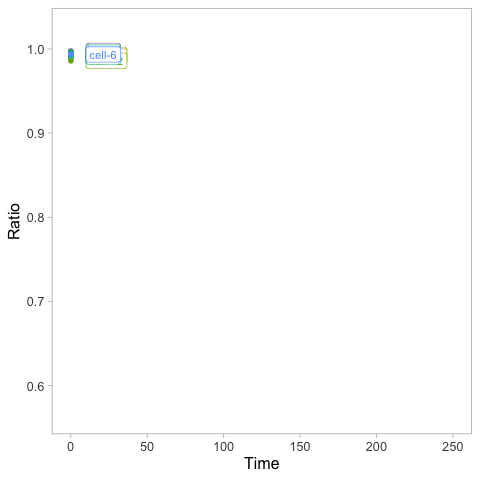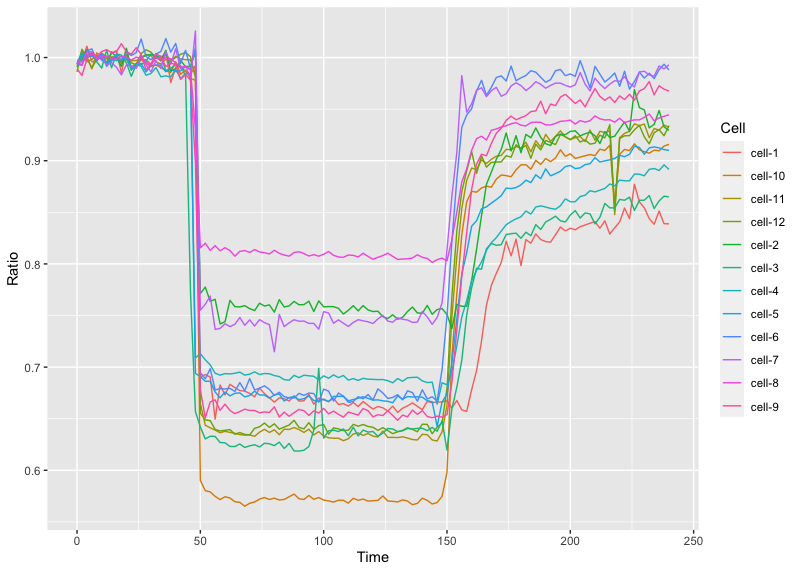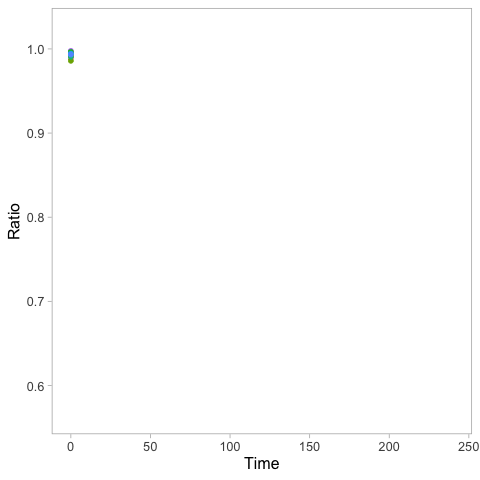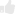# Dynamic display of data with animated plots

Posted by , on 27 April 2020

Data from time-lapse experiments is often displayed in a graph or plot, to visualize the dynamics of biological systems (Goedhart, 2020). Ironically, the perception of the dynamics is largely lost in a static plot. That’s where animated plots come in. Animated plots are a great way to display the dynamics of the underlying data. Below, I provide a walk-through for creating an animated time series using R in Rstudio (footnote 1). The final result looks like this:#### Static plot

The basis for the animation is a static plot. So I start with a brief explanation of how to generate a plot from time-series data (a more detailed explanation of plotting timeseries data can be found in a previous blog). First we load the ggplot2 package that is used for plotting:

`>require(ggplot2)`

Next, we can read the file, which contains the data in a ‘tidy’ format (to transform your own wide, spreadsheet data into tidy format see this blog) and assign it to the dataframe `df_tidy`:

`>df_tidy <- read.csv("https://raw.githubusercontent.com/JoachimGoedhart/Animate-Labeled-TimeSeries/master/FRET-ratio-tidy.csv")`

We can generate an ordinary line plot from the dataframe `df_tidy`:

`>ggplot(df_tidy, aes(x=Time, y=Ratio, color=Cell)) + geom_line()`

This is the result:#### Adding dynamics

Animated plots can be generated with the gganimate package and we will be using functions from the magick package for saving GIFs and so both are loaded:

```>require(gganimate)
>require(magick)```

To turn the static plot into an animated plot, we add the function `transition_reveal(Time)` to reveal the data over time:

```>ggplot(df_tidy, aes(x=Time, y=Ratio, color=Cell)) + geom_line() +
transition_reveal(Time)```

Running this command may take some time (at least a minute on my MacBook). Once it’s ready, the animation is shown in the Viewer panel in RStudio. We will discuss how to save the animation in a bit. But first we will beautify the animation by adding a vertical line that runs along:

```>ggplot(df_tidy, aes(x=Time, y=Ratio, color=Cell)) + geom_line() +
geom_vline(aes(xintercept = Time)) + transition_reveal(Time)```

Another option is to add a dot instead of a line to the leading edge to indicate the time:

```>ggplot(df_tidy, aes(x=Time, y=Ratio, color=Cell)) + geom_line() +
geom_point(size=2) + transition_reveal(Time)```

To save the animation, we first store it as a new object called `animated_plot`:

```>animated_plot <- ggplot(df_tidy, aes(x=Time, y=Ratio, color=Cell)) +
geom_line() + geom_point(size=2) + transition_reveal(Time)```

One of the advantages of this step is that we can do some styling of this object. For instance, changing the default R/ggplot2 layout to a more neutral look:

`>animated_plot <- animated_plot + theme_light(base_size = 16)`

Remove the grid:

`> animated_plot <- animated_plot + theme(panel.grid.major = element_blank(), panel.grid.minor = element_blank())`

Remove the legend:

`> animated_plot <- animated_plot + theme(legend.position="none")`

Now we can render the animation and assign it to the object `animation`. The number of frames in the animation can be specified and it makes sense to use the number of time-points (footnote 2).

`>animation <- animate(animated_plot, nframes=70, renderer=magick_renderer())`

The animation can be displayed in the Viewer panel in RStudio by entering its name in the command line:

`>animation`

To save the animation as a GIF in the working directory, run:

`>image_write_gif(animation, 'animation.gif')`

This is the resulting GIF with the animation:Finally, moving labels can be added to the animation:

`>animated_plot <- animated_plot + geom_label(aes(x = Time, y=Ratio, label=Cell), nudge_x = 10, size=4, hjust=0)`

This needs to be rendered again before it can be displayed or saved:

`>animation <- animate(animated_plot, nframes=70, renderer=magick_renderer())`

Saving this as a GIF will give the animation that is shown at the start of this blog.

#### Final words

I like the animated plot as it elegantly displays the dynamics of the data. The animated plot can be combined with a movie of the process. But that will be a topic for a next blog…

A Shout-out to: Thomas Lin Pedersen who is the driving force behind the wonderful gganimate package. The code that was used here is based on this example: https://github.com/thomasp85/gganimate/wiki/Temperature-time-series

#### Footnotes

Footnote 1: If you are familiar with R you may skip the tutorial and try this R-script: https://github.com/JoachimGoedhart/Animate-Labeled-TimeSeries

Footnote 2: The default for generating high-quality GIFs is the Gifski renderer, but I had some issues with the rendering. To use the default type and save enter:

`>animate(animated_plot, 70, renderer = gifski_renderer("animation.gif"))`(7 votes)Loading...

Tags: , , , , , ,
Categories: Education, Research

## Get involved

Create an account or log in to post your story on the Node.

## Sign up for emails

Subscribe to our mailing lists.

## Contact us

Do you have a question or suggestion for the Node?

## We’re hiring: Community Manager at the Node

A multifaceted science communication opportunity centred around your community site

## Have you seen our new topic pages?